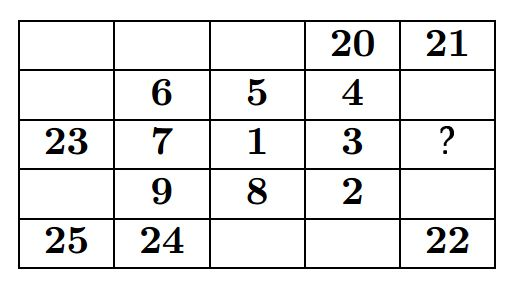# Complete the Square Grid!

Logic Level 2In the diagram given above, the numbers from 1 to 25 are to arranged in a $5 \times 5$ square grid so that each number except 1 and 2, is the sum of two of its neighbours. Some of the numbers have already been filled in. Which number must replace the " $?$ " when the grid is complete.

Note - Numbers in the grid are neighbours if their squares touch along a side or at a corner. For example: the number "1" has eight neighbours, the number "21" has three neighbours.

Bonus - Complete the Grid.

×